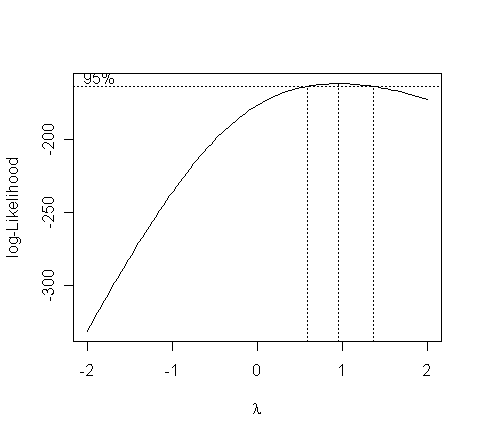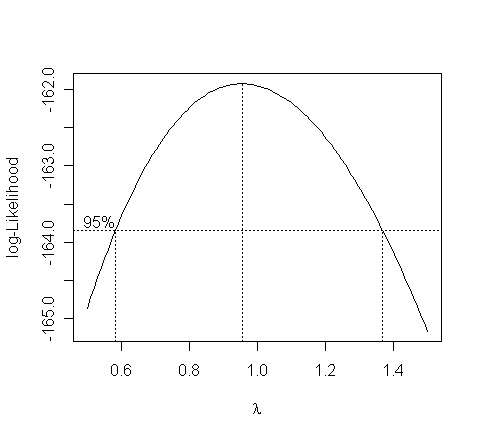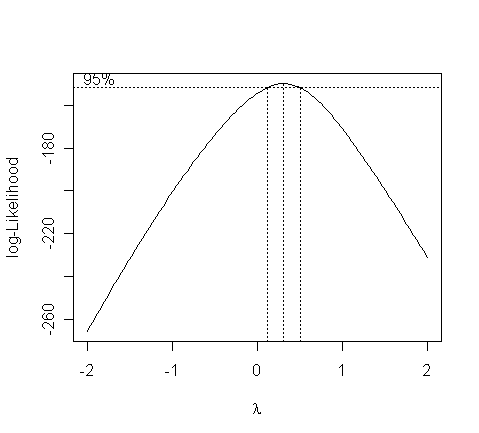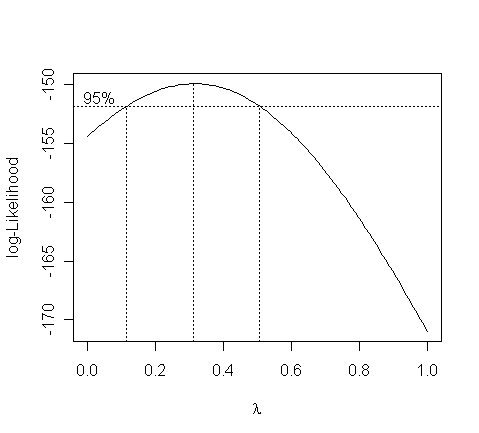# Transforming response

Does the response in the savings data need transformation? You'll need a function from the "MASS" library for performing Box-Cox transformation. Read in the library:

> library(MASS)

Try it out on the savings dataset:

> g <- lm(sav ~ p15 + p75 + inc + gro, data=savings)
> boxcox(g, plotit=T)> boxcox(g, plotit=T, lambda=seq(0.5,1.5,by=0.1))• The confidence interval for lambda is from 0.6 to about 1.4. What do we conclude?

• We can see that there is no good reason to transform.

Now consider the Galapagos data analyzed earlier:

> boxcox(gg, plotit=T)> boxcox(gg, lambda=seq(0.0,1.0,by=0.05), plotit=T)• The confidence interval for lambda is from 0.1 to about 0.5. What do we conclude?

• We see that perhaps a cube-root transformation might be best here.

• A square root is also a possibility as this falls just within the confidence intervals. Certainly there is a strong need to transform.

# Transforming predictors

Let's see if the gro variable in the savings dataset needs transformation:

> g <- lm(sav ~ p15 + p75 + gro + inc, data=savings)
> g2 <- update(g, . ~ . + I(gro*log(gro))) # Add gro*log(gro) to the model
> summary(g2)

Coefficients:

Estimate Std. Error t value Pr(>|t|)

(Intercept)       23.7631766  8.6192503   2.757  0.00846 **

p15               -0.4026101  0.1545631  -2.605  0.01249 *

p75               -1.3604915  1.1258041  -1.208  0.23332

gro                1.6904514  1.2190155   1.387  0.17251

inc               -0.0004147  0.0009326  -0.445  0.65874

I(gro * log(gro)) -0.4675886  0.4392646  -1.064  0.29292

---

Residual standard error: 3.797 on 44 degrees of freedom

Multiple R-Squared: 0.3551,     Adjusted R-squared: 0.2818

F-statistic: 4.845 on 5 and 44 DF,  p-value: 0.001291

• Examine the coefficient of gro*log(gro) - what should we conclude?

Now see if p15 should be transformed.

> g3 <- update(g, . ~ . + I(p15*log(p15))) ;  summary(g3)